### Home > CALC > Chapter Ch2 > Lesson 2.2.1 > Problem2-55

2-55.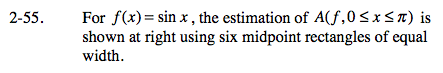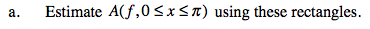Base of each rectangle:

$\Delta x=\frac{\pi }{6}$

Height of each rectangle is determined by the function: f(x) = sinx evaluated at each midpoint.

Let's examine one of the rectangles, the smallest one:

$\text{Midpoint of smallest rectangle: }x= \frac{1}{2}\left ( \frac{\pi }{6} \right )=\frac{\pi }{12}$

$\text{Height of smallest rectangle: }y= \text{sin}\frac{\pi }{12}$

$\text{Area of smallest rectangle: }A= bh=\left ( \frac{\pi }{6} \right )\left ( \frac{\pi }{12} \right )=\left (\frac{\pi }{72} \right )$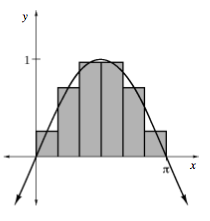Two medium sized rectangles with midpoints at ____ and ____.
Two large rectangles with midpoints at ____ and ____.
Find the area of all six rectangles.

Notice there are two small rectangles with midpoints at

$\frac{\pi }{12}\text{ and } \frac{11\pi }{12}.$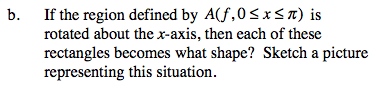Imagine a barbell with six cylindrical weight: two small, two medium and two large. Each cylinder has a thickness (or height) of

$\frac{\pi }{6},$

but their circular bases vary in sizes. The radius of each circular base is determined by the function f(x) = sin x evaluated at

$x=\frac{\pi }{12}, \frac{3\pi }{12}, \frac{5\pi }{12}, \frac{7\pi }{12}, \frac{9\pi }{12}, \frac{11\pi }{12}.$

The smallest cylinders are on the outside, then medium then large.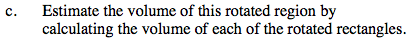Because of symmetry, you can evaluate the volume of three of the cylinders and then double the result.

Volume of a cylinder: V= πr²h = πr²(Δx)